In :
import numpy as np
import matplotlib.pyplot as plt
import pandas as pd

In :
%matplotlib inline

In :
plt.plot(np.arange(10));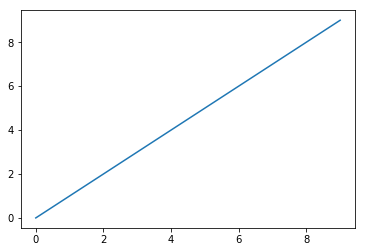## Figures and Subplots¶

In :
fig = plt.figure()

<Figure size 432x288 with 0 Axes>

figsize gaurantees the figure with have a size and aspect ratio

You can't make a plot with a blank Figure, you have to create one or more subplots

In :
ax1 = fig.add_subplot(2, 2, 1)


This means the figure should be 2 x 2 and we are selecting the first of 4 subplots

In :
ax2 = fig.add_subplot(2, 2, 2)

In :
fig

Out: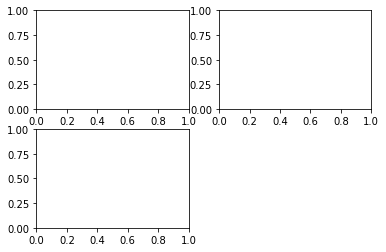Issuing a plotting command will draw on the last figure and subplot used (apparently, but not in this notebook)

In :
plt.plot([1.5, 3.5, -2, 1.6]);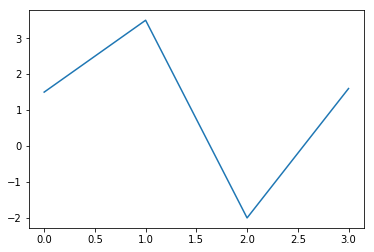In :
plt.plot(np.random.randn(50).cumsum(), 'k--');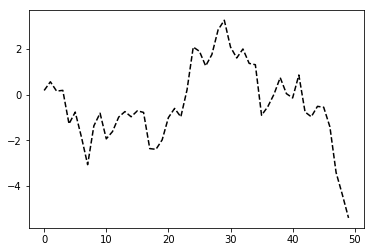The k-- parameter is a style option telling matplotlib to print a dashed line

The object returned is a AxesSubPlot which you can plot on a subplot using it's instance methods

In :
_ = ax1.hist(np.random.randn(100), bins=20, color='k', alpha=0.3)

In :
fig

Out: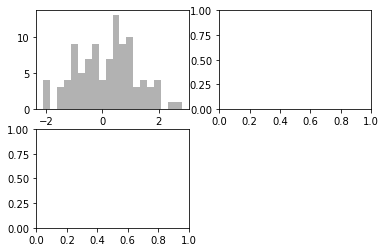In :
ax2.scatter(np.arange(30), np.arange(30) + 3 * np.random.randn(30))
fig

Out: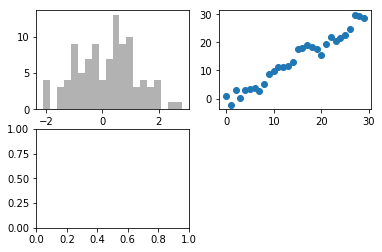Since creating subplots is such a common task, there is a convenient method plt.sublots that creates a new figure and returns the subplot objects

In :
fig, axes = plt.subplots(2, 3)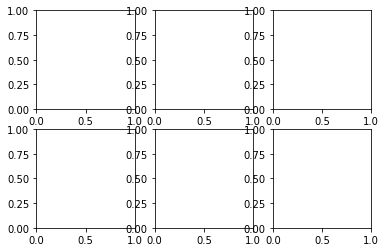Axes are indexed just like a two dimensional array

In :
axes[0, 1]

Out:
<matplotlib.axes._subplots.AxesSubplot at 0x114b91e10>

By default matplotlib adds space around plots relative to height and width of the subplots. It can be adjusted with subplots_adjust

subplots_adjust(left=None, botttom=None, right=None, top=None, wspace=None, hspace=None)

wspace and hspace control the percentage of the figure to be used for spacing

In :
fig.subplots_adjust(wspace=0, hspace=0)
fig

Out: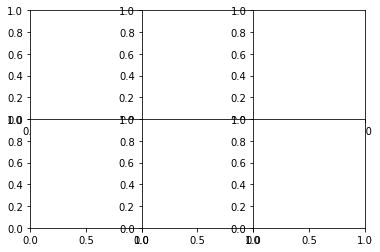There is some overlapping of labels, which matplot does not fix for you, you must must fix there sourself.

### Colours, Markers and Line Styles¶

the plot function accepts arrays of x and y coordinates and optional kwargs for colour and line style

ax.plot(x, y, 'k--')

More explicitly

ax.plot(x, y, linestyle='--', color='g')

Color can also be a hex RGB colour

Line plots have markers to indicate the data points, sometimes it is unclear where the points lie.

In :
plt.plot(np.random.randn(30).cumsum(), 'ko--');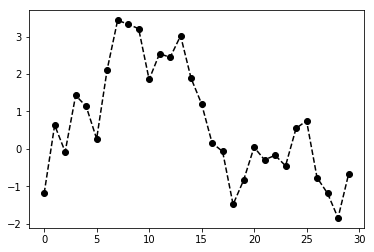More explicitly (and explicit is better than implicit)

In :
plt.plot(np.random.randn(30).cumsum(), color='k', linestyle='dashed', marker='o');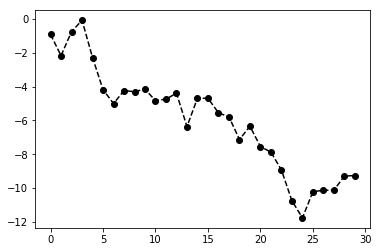You can draw the line in a different way:

In :
data = np.random.randn(30).cumsum()
plt.plot(data, 'k--', label='Default')
plt.plot(data, 'k--', drawstyle='steps-post', label='steps-post')
plt.legend(loc='best');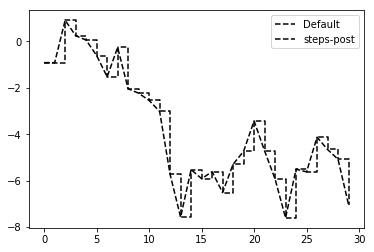### Ticks, Labels and Legends¶

There are 2 ways to plot:

• the procedural pyplot interface
• the object oriented native matplotlib API

The pyplot interface has methods xlim, xticks and xticklabels. These control plot range, tick locations and tick labels.

plt.xlim() returns the current x axis plotting range. Called with parameters, sets the range: plt.xlim([0, 10])

The corresponding api methods are ax.get_xlim and ax.get_ylim

In :
fig = plt.figure(); ax = fig.add_subplot(1, 1, 1)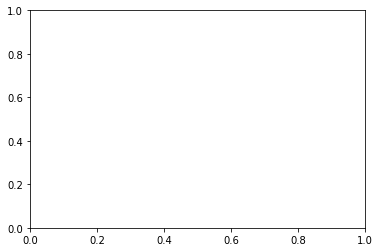In :
ax.plot(np.random.randn(1000).cumsum())
fig

Out: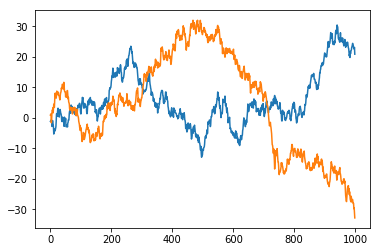set_xticks tells matplotlib where to place the ticks on the x axis. set_xticklabels sets the labels.

In :
ticks = ax.set_xticks([0, 250, 500, 750, 1000])
labels = ax.set_xticklabels(['one', 'two', 'three', 'four', 'five'], rotation=30, fontsize='small')


set_title sets teh title of the subplot. set_xlabels sets the label for the x axis.

In :
ax.set_title('My First Matplot Plot')
ax.set_xlabel('Stages')
fig

Out: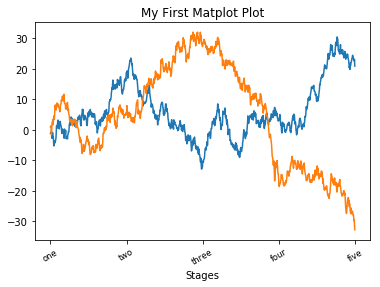The easiest thing to do is set the label of each part of the plot

In :
fig = plt.figure(); ax = fig.add_subplot(1, 1, 1)
ax.plot(np.random.randn(1000).cumsum(), 'k', label='one')
ax.plot(np.random.randn(1000).cumsum(), 'k--', label='two')
ax.plot(np.random.randn(1000).cumsum(), 'k.', label='three')
ax.legend(loc='best');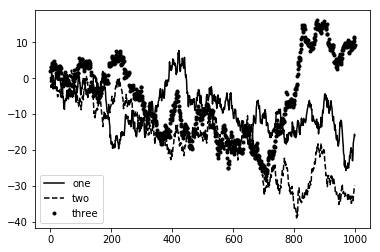You can use ax.legend() or plt.legend() to add the legend. The kwarg loc tells where to put the legend (best is a good choice). To exlcude from the legend, you can set label='__no_legend__'

### Annotations¶

Text, arrows and other shapes. Using teh text, arrow and annotate functions

In :
from datetime import datetime

Out:
SPX
1990-02-01 328.79
1990-02-02 330.92
1990-02-05 331.85
1990-02-06 329.66
1990-02-07 333.75
In :
spx = data['SPX']

In :
fig = plt.figure(); ax = fig.add_subplot(1, 1, 1)
spx.plot(ax=ax, style='k-');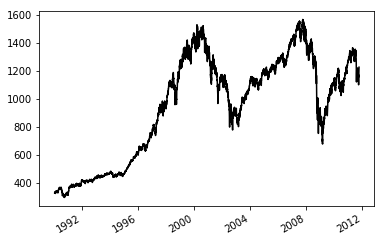In :
crisis_data = [
(datetime(2007, 10, 11), 'Peak of the Bull Market'),
(datetime(2008, 3, 12), 'Bear Sterns Fails'),
(datetime(2008, 9, 15), 'Lehman Bankrupcy')
]

In :
for date, label in crisis_data:
ax.annotate(label, xy=(date, spx.asof(date) + 50), xytext=(date, spx.asof(date) + 200),
arrowprops=dict(facecolor='black'), horizontalalignment='left', verticalalignment='top')

In :
# Zoom in on 2007 - 2010
ax.set_xlim(['1/1/2007', '1/1/2011'])
ax.set_ylim([600, 1800])

Out:
(600, 1800)
In :
ax.set_title('Important dates in 2008-2009 financial crisis')

Out:
Text(0.5, 1.0, 'Important dates in 2008-2009 financial crisis')
In :
fig

Out: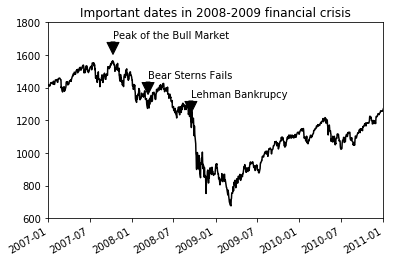Drawing shapes requires more care, matplotlib has objects that represent many common shapes, reffered to as patches. Some like Rectangle and Circle are found in matplotlib.pyplot, the full set is located in matplotlib.patches

Adding a shape to a plot, you create the patch object shp and add it to the subplot by calling ax.add_patch(shp)

In :
fig = plt.figure(); ax = fig.add_subplot(1, 1, 1);
rect = plt.Rectangle((0.2, 0.75), 0.4, 0.15, color='k', alpha=0.3)
circ = plt.Circle((0.7, 0.2), 0.15, color='b', alpha=0.3)
pgon = plt.Polygon([[0.15, 0.15], [0.35, 0.4], [0.2, 0.6]], color='g', alpha=0.5)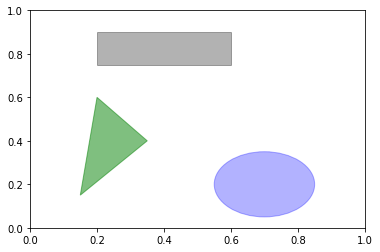### Saving Plots to File¶

The active figure can be saved with plt.savefig

To save an svg:

In :
fig.savefig('shapes.svg')


The file type is inferred from the file extensions

In :
fig.savefig('shapes.png', dpi=400, bbox_inches='tight')


savefig doesn't have to write to disk, it can also write to any file-like object such as BytesIO

In :
from io import BytesIO
buffer = BytesIO()
plt.savefig(buffer)

<Figure size 432x288 with 0 Axes>
In :
plot_data = buffer.getvalue()


This is useful for serving dynamically-generated images over the web.

### Matplotlib Configuration¶

Has colour schemes and defaults geared towards publications. Nearly all of this behaviour can be customised. For example you can set a global figure size:

In :
plt.rc('figure', figsize=(10, 5))


The first argument to rc is the component you want to customise.

In :
font_options = {'family': 'monospace', 'weight': 'bold', 'size': 15}
plt.rc('font', **font_options)


There is matplotlibrc file in: matplotlib/mpl-data which you can put in yourt home directory and it will be loaded each time you run matplotlib.

## Plotting with Pandas and Seaborn¶

matplotlib can be fairly low level. You assemble the plot from it's base components: line, bar, box, scatter, legend, title, tick labels and others. Pandas has uilt-in methods which simplify visualisation creation.

### Line Plots¶

Series and Dataframes have the plot function for basic plots

In :
s = pd.Series(np.random.randn(10).cumsum(), index = np.arange(0, 100, 10))
s.plot()

Out:
<matplotlib.axes._subplots.AxesSubplot at 0x116f9e8d0>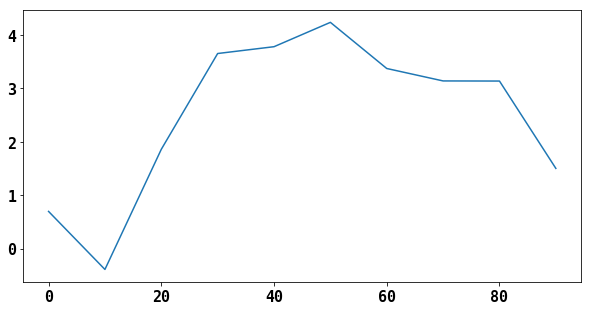The series's index is set on the x-axis. You can exclude it using use_index=False. The ticks and limits can be adjusted using xlim and xticks

It accepts an ax attribute to send the plot to a specific subplot object

In :
df = pd.DataFrame(np.random.randn(10, 4).cumsum(0), columns=['a', 'b', 'c', 'd'], index=np.arange(0, 100, 10))
df.plot();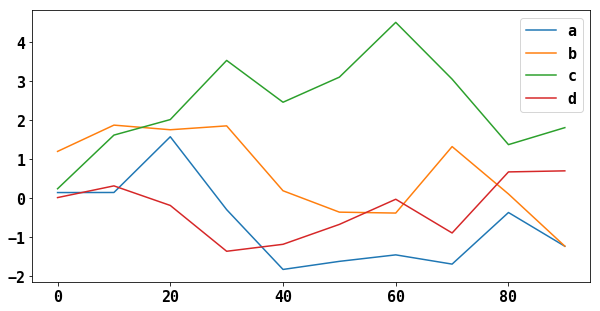### Bar Plots¶

plot.bar() and plot.barh() make vertical and horizontal bar plots. In this case the index will be used as the x (bar) or y (barh) ticks

In :
fig, axes = plt.subplots(2, 1)
data = pd.Series(np.random.rand(16), index=list('abcdefghijklmnop'))
data.plot.bar(ax=axes, color='k', alpha=0.7)

Out:
<matplotlib.axes._subplots.AxesSubplot at 0x116c6c128>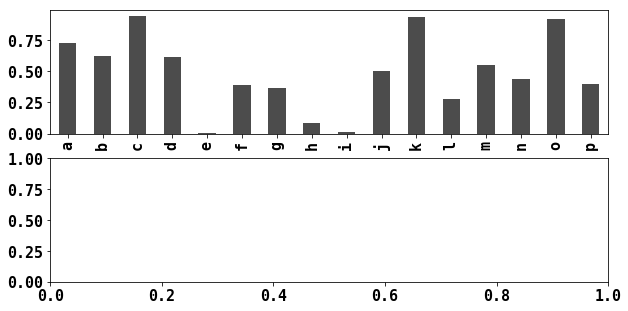In :
data.plot.barh(ax=axes, color='k', alpha=0.7)
fig

Out: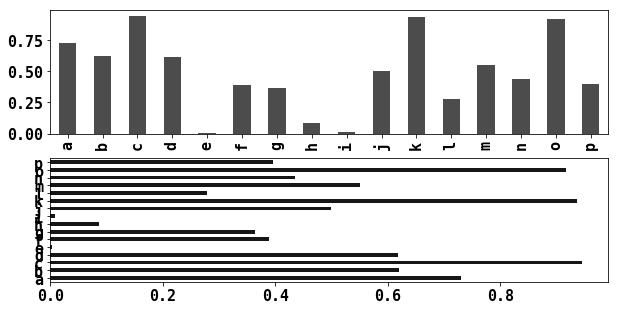With a DataFrame, group row values together

In :
df = pd.DataFrame(
np.random.rand(6, 4),
index=['one', 'two', 'three', 'four', 'five', 'six'],
columns=pd.Index(['A', 'B', 'C', 'D'], name='Genus')
)
df

Out:
Genus A B C D
one 0.291024 0.425924 0.832796 0.027127
two 0.680509 0.211131 0.971022 0.489830
three 0.751700 0.332034 0.621238 0.433753
four 0.832437 0.331309 0.959043 0.274794
five 0.976971 0.416167 0.960919 0.741820
six 0.248145 0.930182 0.927123 0.958313
In :
df.plot.bar();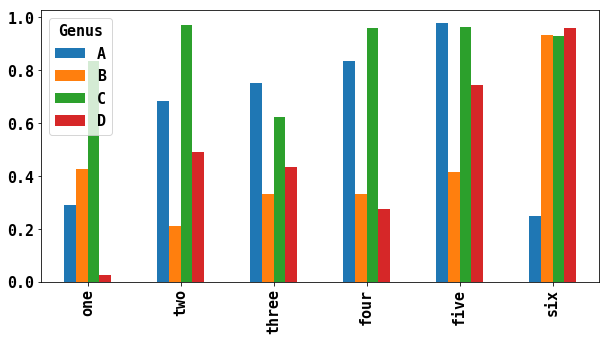Not the plot name, Genus is used as the title of the legend

Stacked bar plots are created by passing stacked=True, resulting in each row being stacked together

In :
df.plot.barh(stacked=True, alpha=0.5);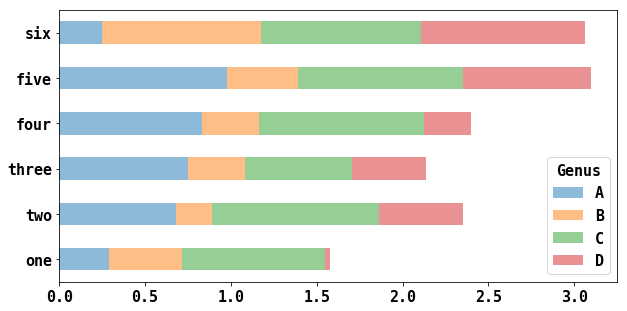A useful recipe for bar plots is to visualise a series's value frequency using: s.value_counts().plot.bar()

In :
tips = pd.read_csv('tips.csv')

Out:
total_bill tip smoker day time size
0 16.99 1.01 No Sun Dinner 2
1 10.34 1.66 No Sun Dinner 3
2 21.01 3.50 No Sun Dinner 3
3 23.68 3.31 No Sun Dinner 2
4 24.59 3.61 No Sun Dinner 4
In :
party_counts = pd.crosstab(tips['day'], tips['size'])
party_counts

Out:
size 1 2 3 4 5 6
day
Fri 1 16 1 1 0 0
Sat 2 53 18 13 1 0
Sun 0 39 15 18 3 1
Thur 1 48 4 5 1 3

There are not many 1 and 6 party tables. Normalise so each row sums to 1

In :
party_counts = party_counts.loc[:, 2:5]

In :
party_counts

Out:
size 2 3 4 5
day
Fri 16 1 1 0
Sat 53 18 13 1
Sun 39 15 18 3
Thur 48 4 5 1
In :
party_pcts = party_counts.div(party_counts.sum(1), axis=0)
party_pcts

Out:
size 2 3 4 5
day
Fri 0.888889 0.055556 0.055556 0.000000
Sat 0.623529 0.211765 0.152941 0.011765
Sun 0.520000 0.200000 0.240000 0.040000
Thur 0.827586 0.068966 0.086207 0.017241
In :
party_pcts.plot.bar();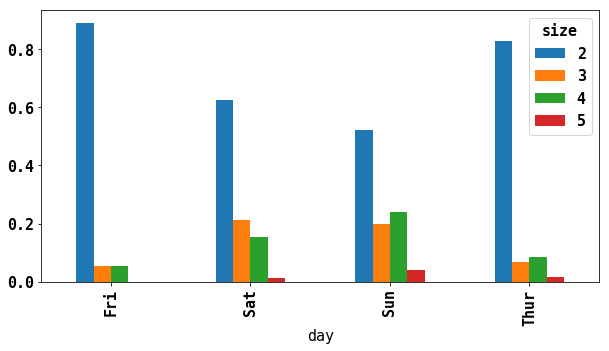With data requiring aggregation and summarisation before making a plot, using the seaborn package can make things simpler.

In :
import seaborn as sns
tips['tip_pct'] = tips['tip'] / (tips['total_bill'] - tips['tip'])

Out:
total_bill tip smoker day time size tip_pct
0 16.99 1.01 No Sun Dinner 2 0.063204
1 10.34 1.66 No Sun Dinner 3 0.191244
2 21.01 3.50 No Sun Dinner 3 0.199886
3 23.68 3.31 No Sun Dinner 2 0.162494
4 24.59 3.61 No Sun Dinner 4 0.172069
In :
sns.barplot(x='tip_pct', y='day', data=tips, orient='h');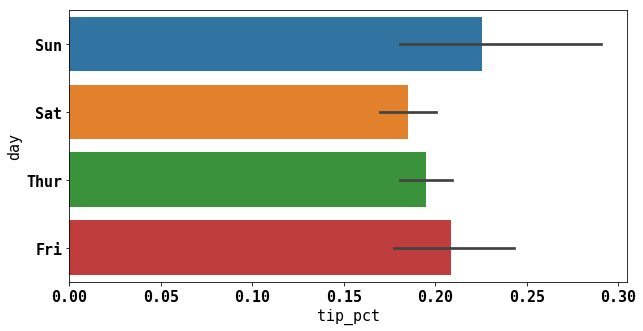Plotting in seaborn takes the data argument. The bloack lines represent the 95% confidence level.

seaborn has the hue option to split up against another categorical value:

In :
sns.barplot(x='tip_pct', y='day', data=tips, hue='time', orient='h');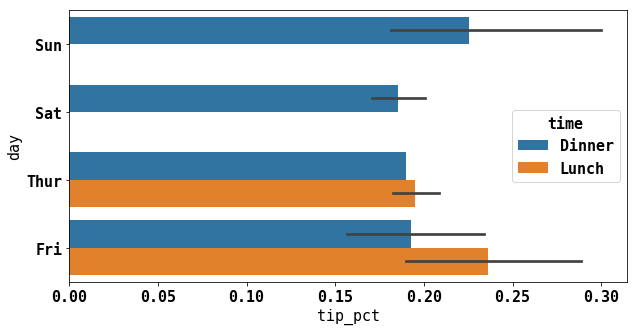You can set different appearance attributes with sns.set

In :
sns.set(style='whitegrid')

In :
sns.barplot(x='tip_pct', y='day', data=tips, hue='time', orient='h');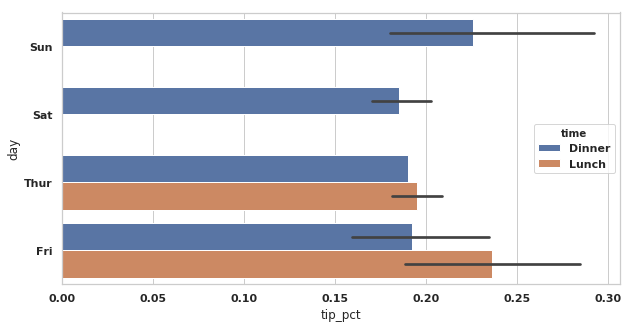### Histograms and Density Plots¶

A histogram is like a bar plot that gives a display of value frequency. The data points are split into discrete evenly spaced bins.

In :
tips['tip_pct'].plot.hist(bins=50);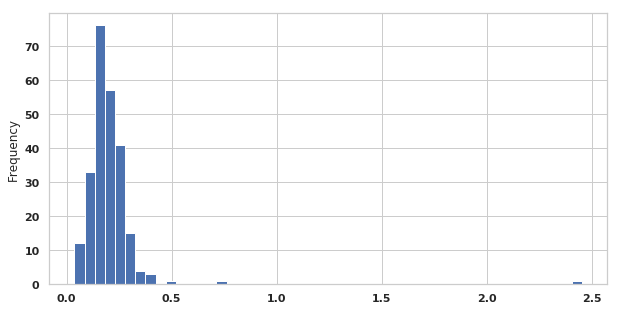A related plot type is a density plot, which is an estimate of continuous probability distribution that might have enerated the observed data. They are also known as KDE (Kernal Density Estimate) plots.

In :
tips['tip_pct'].plot.density();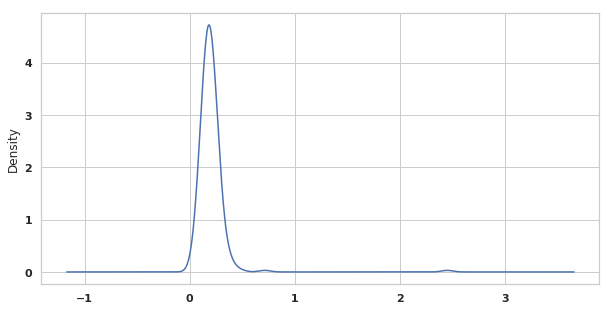Seaborn makes it even easier with it's distplot method, which plots a histogram and density plot simulataneously. Consider a bimodal distribution consisting of draws from two differnt standard normal distributions:

In :
comp1 = np.random.normal(0, 1, size=200)
comp2 = np.random.normal(10, 2, size=200)
values = pd.Series(np.concatenate([comp1, comp2]))
sns.distplot(values, bins=100, color='k');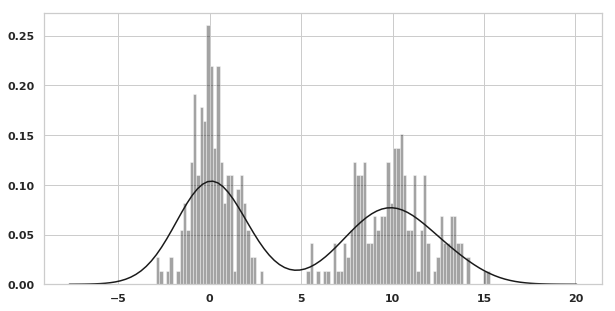### Scatter or Point Plots¶

Useful for examing the relationship between 2 one-dimensional data series.

In :
macro = pd.read_csv('macrodata.csv')

Out:
year quarter realgdp realcons realinv realgovt realdpi cpi m1 tbilrate unemp pop infl realint
0 1959.0 1.0 2710.349 1707.4 286.898 470.045 1886.9 28.98 139.7 2.82 5.8 177.146 0.00 0.00
1 1959.0 2.0 2778.801 1733.7 310.859 481.301 1919.7 29.15 141.7 3.08 5.1 177.830 2.34 0.74
2 1959.0 3.0 2775.488 1751.8 289.226 491.260 1916.4 29.35 140.5 3.82 5.3 178.657 2.74 1.09
3 1959.0 4.0 2785.204 1753.7 299.356 484.052 1931.3 29.37 140.0 4.33 5.6 179.386 0.27 4.06
4 1960.0 1.0 2847.699 1770.5 331.722 462.199 1955.5 29.54 139.6 3.50 5.2 180.007 2.31 1.19
In :
data = macro[['cpi', 'm1', 'tbilrate', 'unemp']]
transdata = np.log(data).diff().dropna()
transdata[-5:]

Out:
cpi m1 tbilrate unemp
198 -0.007904 0.045361 -0.396881 0.105361
199 -0.021979 0.066753 -2.277267 0.139762
200 0.002340 0.010286 0.606136 0.160343
201 0.008419 0.037461 -0.200671 0.127339
202 0.008894 0.012202 -0.405465 0.042560
In :
sns.regplot('m1', 'unemp', data=transdata);

/Library/Frameworks/Python.framework/Versions/3.6/lib/python3.6/site-packages/scipy/stats/stats.py:1713: FutureWarning: Using a non-tuple sequence for multidimensional indexing is deprecated; use arr[tuple(seq)] instead of arr[seq]. In the future this will be interpreted as an array index, arr[np.array(seq)], which will result either in an error or a different result.
return np.add.reduce(sorted[indexer] * weights, axis=axis) / sumval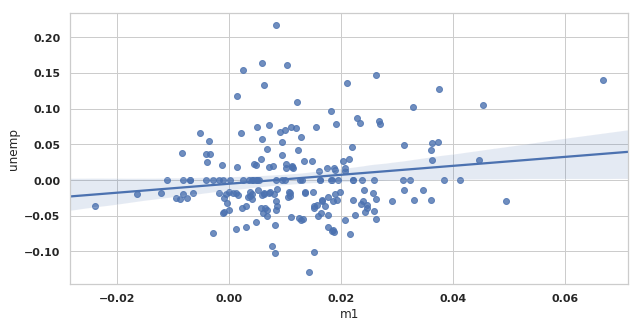In exploratory data analysis it is important to look at scatter plots among groups of variables, known as a scatter plot matrix

Making such plot from scratch is a bit of work, so seaborn has the pairplot method.

In :
sns.pairplot(transdata, diag_kind='kde', plot_kws={'alpha': 0.2})

Out:
<seaborn.axisgrid.PairGrid at 0x11c3d6cf8>